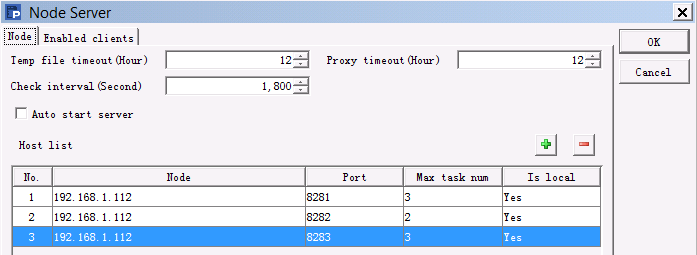# 枚举轴

 A 1 =canvas() 2 =demo.query("select * from FRUITS") 3 =A1.plot("BackGround") 4 =A1.plot("EnumAxis","name":"x","categories":A2.(NAME)) 5 =A1.plot("NumericAxis","name":"y","location":2) 6 =A1.plot("Column","axis1":"x","data1":A2.(NAME),"axis2":"y","data2": A2.(UNITPRICE)) 7 8 =A1.draw@p(350,200)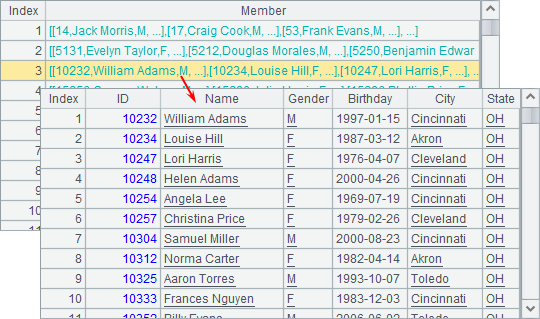A3绘制白色背景；A4中设定横轴x为枚举轴，并设定分类序列为水果名称构成的序列；A5中设定纵轴y为数值轴，自动计算值域；A6中绘制柱图，绘制的逻辑坐标为水果名称和水果单价。A8绘制结果如下：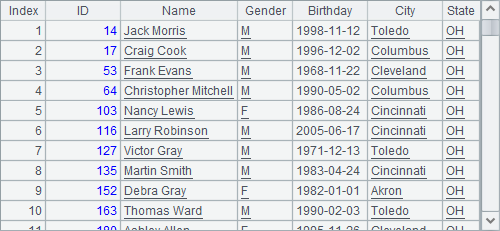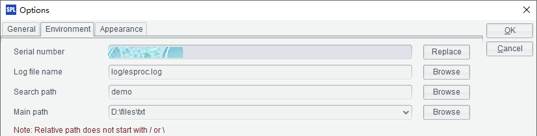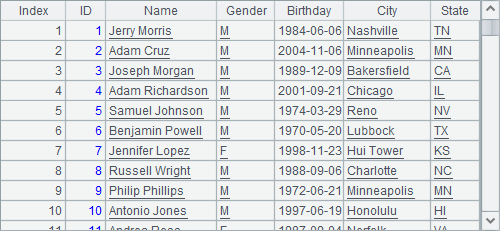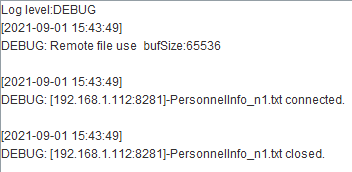A 1 =canvas() 2 =demo.query("select * from GYMSCORE") 3 =A1.plot("BackGround") 4 =A1.plot("EnumAxis","name":"x") 5 =A1.plot("NumericAxis","name":"y","location":2) 6 =A1.plot("Column","axis1":"x","data1":A2.(EVENT+","+NAME),"axis2":"y","data2":A2.(SCORE)) 7 8 =A1.draw@p(450,250)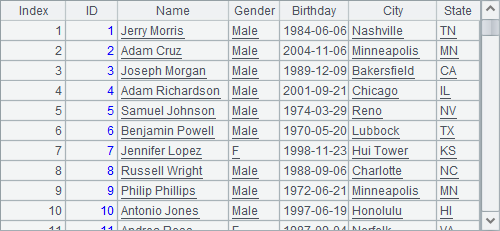A3绘制白色背景；A4中设定横轴x为枚举轴，自动分析数据；A5中设置纵轴y为数值轴；A6中绘制柱图。同时使用分类和系列时，枚举轴上的逻辑坐标需要包含分类和系列的信息，将分类和系列的值用逗号分隔开，如"Vault,Becky Downie"

A8中绘图结果如下：